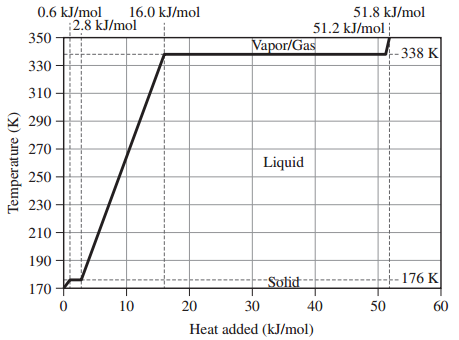# Problem: Assume that the values given here for 1 mole of methanol are constant over the relevant temperature ranges.Melting Point176 KBoiling Point338 KHfus2.2 kJ/mol KHvap35.2 kJ/mol KCs,solid105 J/mol KCs,liquid81.3 J/mol KCs,gas48 J/mol KGiven the heating curve for 1 mole of methanol beginning at 170 K and ending at 350 K answer the following questions:What is the value of qsolid for methanol?

🤓 Based on our data, we think this question is relevant for Professor Nishida's class at WSU.

###### FREE Expert Solution

Given the heating curve for 1 mole of methanol, we are asked to calculate qsolid for methanol.

Recall: In Heating curves, we have the representation of the amount of heat absorbed during phase changes. As the reaction progress, a phase change or temperature change can occur.

During a phase change,

• Temperature is constant
• Avg. Kinetic Energy is constant
• Heat is transferred into potential energy

During a temperature change.

• Heat energy is converted into Kinetic Energy
• Increase in temperature causes increase in Kinetic Energy
• Decrease in temperature causes decrease in Kinetic Energy###### Problem Details
Assume that the values given here for 1 mole of methanol are constant over the relevant temperature ranges.

Melting Point

 176 K

Boiling Point

 338 K

Hfus

 2.2 kJ/mol K

Hvap

 35.2 kJ/mol K

Cs,solid

 105 J/mol K

 Cs,liquid

 81.3 J/mol K

 Cs,gas

48 J/mol K

Given the heating curve for 1 mole of methanol beginning at 170 K and ending at 350 K answer the following questions:What is the value of qsolid for methanol?